## You have 80 minutes to answer 54 questions.

Because of this, Section 1 is the most time-pressured section of the NSAA. This section tests GCSE biology, chemistry, physics and maths. The questions can be quite difficult and it’s easy to get bogged down. However, it’s possible to rapidly improve if you prepare correctly so it’s well worth spending time on it.

As part of section 1, you have to pick two section from biology, chemistry, physics or advanced maths/physics. In most cases it will be immediately obvious to you which section will suit you best. Generally, applicants for physical natural sciences will choose physics/maths whilst those for biological sciences will choose the biology and chemistry. However, like the natural sciences tripos, this is by no means a hard and fast rule – its extremely important that choose the section you want to do ahead of time so that you can focus your preparation accordingly.

If you’re unsure which to pick, review the content of each section and try out some questions so you can get a feel for the style and difficulty. This table shows what the general questions are like for each subject.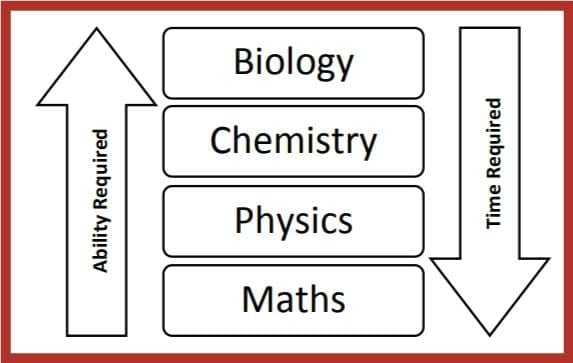### The available sections

Required: Mathematics

Each part has 18 multiple choice questions. There is no negative marking so you should attempt every question in the three parts that you choose. Each question is worth one mark.

Maths is the only required section in the NSAA. Because of this, being confident with Maths is extremely important in the NSAA. We find that students improving their numerical and algebraic skills usually result in massive improvements in both their section 1 and 2 scores. Remember that maths in section 1 not only comes up in the maths questions but also in physics (manipulating equations and standard form) and chemistry (mass calculations). Thus, if you find yourself consistently running out of time in section 1, spending a few hours on brushing up your basic maths skills may do wonders for you.

### Section 1A: Maths

NSAA Maths questions are designed to be time-draining – if you find yourself consistently not finishing, it might be worth leaving the maths (and probably physics) questions until the very end. Good students sometimes have a habit of making easy questions difficult; remember that section 1A is pitched at GCSE level so you are not expected to know or use calculus or advanced trigonometry in it.

These are the formulas you must know: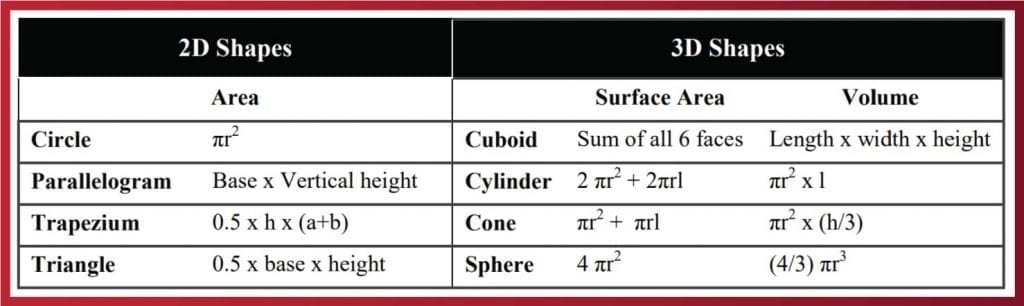Other topics that you should be familiar with include Quadratic Formula and Completing the square. Even students studying A2 maths can struggle with these topics because sometimes they are glossed over at school.

#### Here’s an example of a maths question that you could see on the NSAA. This one is all about probability (you can find the answer here):

Give it a shot and see how you do by checking the worked solution at the end. Our Ultimate NSAA Guide has worked solutions to 300 questions just like this! This is provided with our Natural Science Programme >>>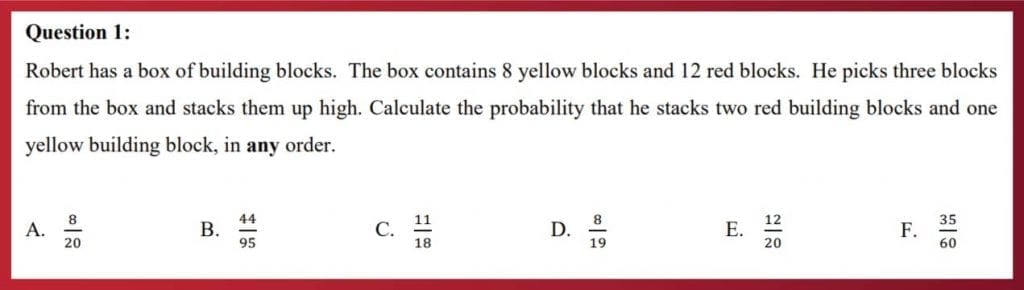### Section 1B: Physics

Physics Questions in the NSAA are challenging as they frequently require you to make leaps in logic and calculations. Thus, before you go any further, ensure you have a firm understanding of the major principles and are confident with commonly examined topics like Newtonian mechanics, electrical circuits and radioactive decay as you may not have covered these at school depending on the specification you did.

The majority of Physics questions require a fair bit of Maths – another reason to brush up on your Maths! Most questions require two step calculations. Here’s a worked example Physics question with two ways of answering the question;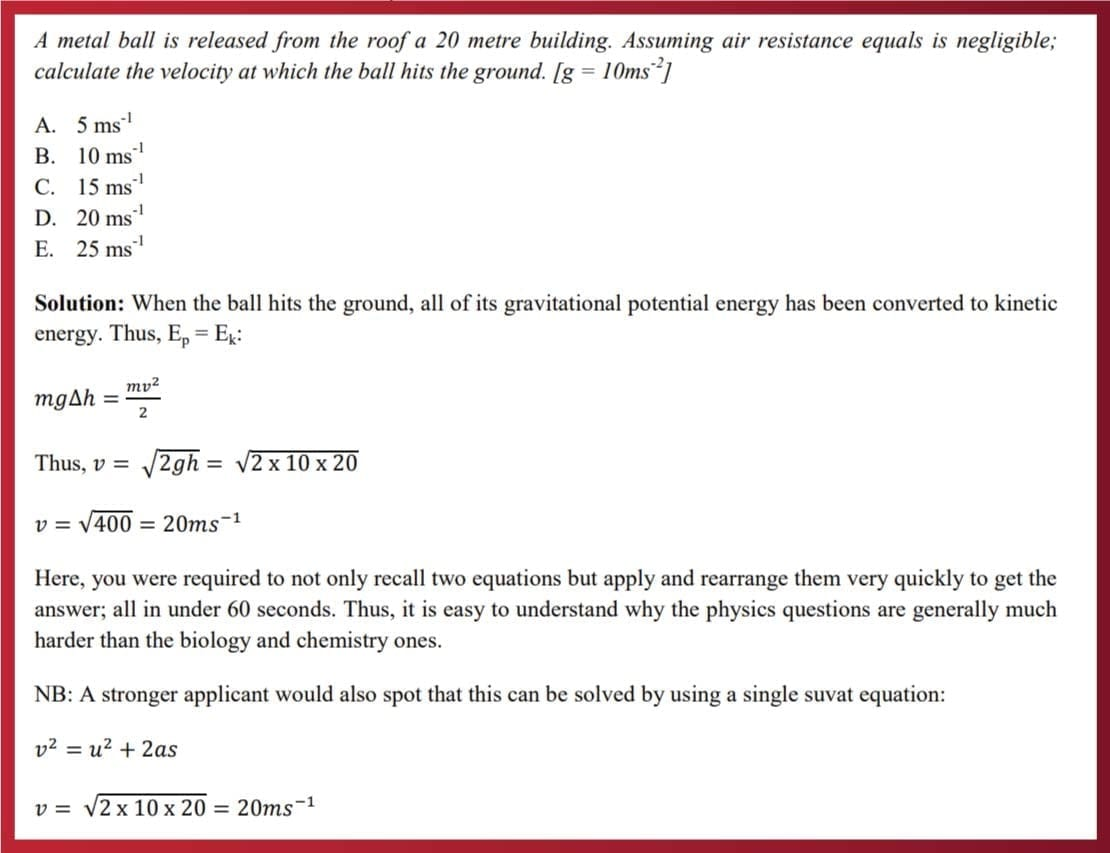### Section 1C: Chemistry

Most students don’t struggle with NSAA chemistry – however, there are certain questions that even good students tend to struggle with under time pressure e.g. balancing equations and mass calculations. It is essential that you’re able to do these quickly as they take up by far the most time in the chemistry questions.

#### Balancing equations

For some reason, most students are rarely shown how to formally balance equations – including those studying it at A-level. Balancing equations intuitively or via trial and error will only get you so far in the NSAA as the equations you’ll have to work with will be fairly complex.

To avoid wasting time, it’s essential that you learn a method that solves these questions in less than 60 seconds consistently. Consider this example below;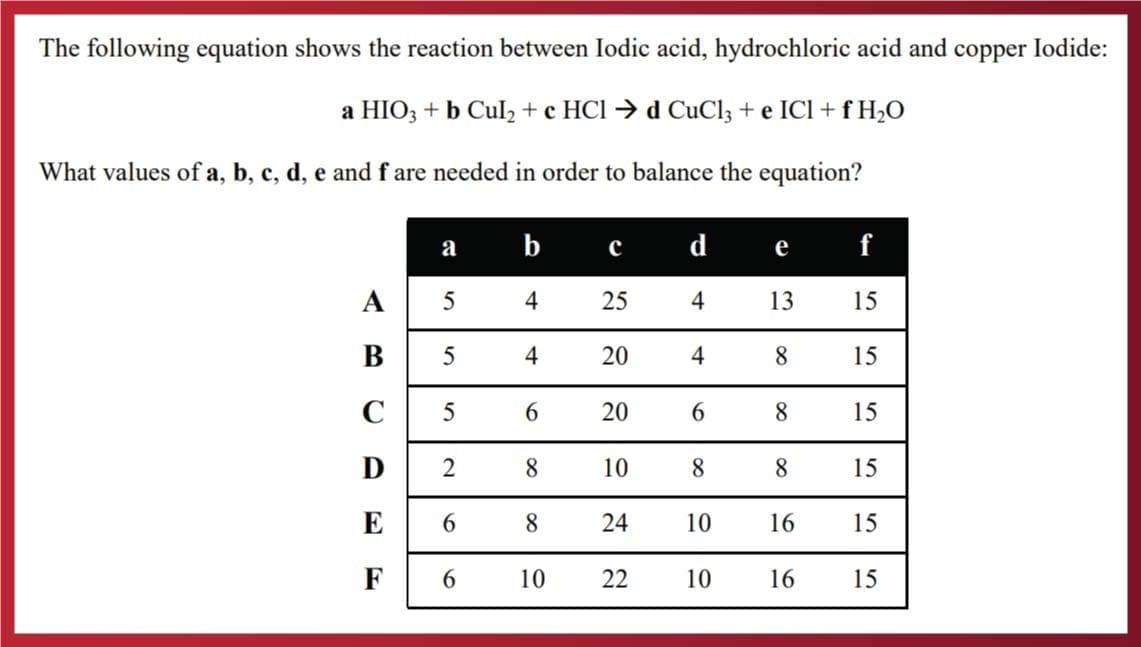The Method

Step 1: Pick an element and see how many atoms there are on the left and right sides.
Step 2: Form an equation to represent this. For Cu: b = d
Step 3: See if any of the answer options don’t satisfy b=d. In this case, for option E, b is 8 and d is 10. This allows us to eliminate option E.

Once you’ve eliminated as many options as possible, go back to step 1 and pick another element.
For Hydrogen (H): a + c = 2f

Then see if any of the answer options don’t satisfy a + c = 2f.

• Option A: 5 + 25 is equal to 2 x 15
• Option B: 5 + 20 is not equal to 2 x 15
• Option C: 5 + 20 is not equal to 2 x 15
• Option D: 2 + 10 is not equal to 2 x 15

This allows us to eliminate option B, C & D. Since E was eliminated earlier, A is the only possible solution. This method works best when you get given a table above as this allows you to quickly eliminate options. However, it is still a viable method even if you don’t get this information.

### The Maths Answer – did you get it right?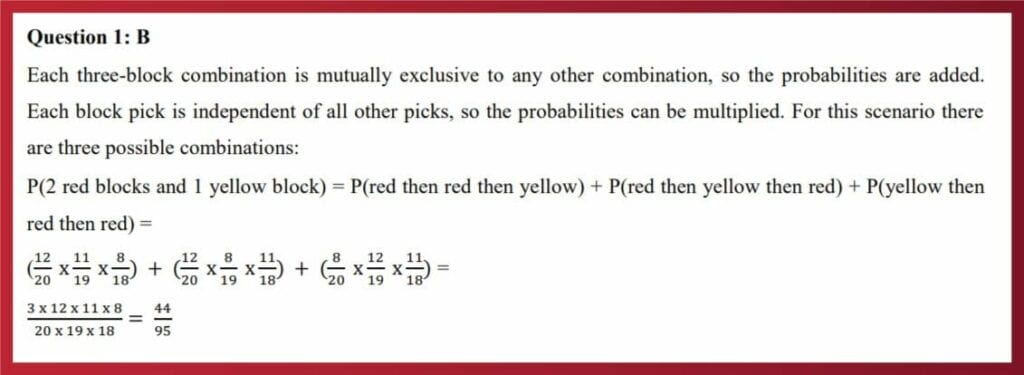#### Natural Sciences at Cambridge is highly competitive due to the unique nature of the course. We can triple your chances of success through one-to-one tuition, group sessions, interview technique and more.

DISCOVER THE NSAA PROGRAMME